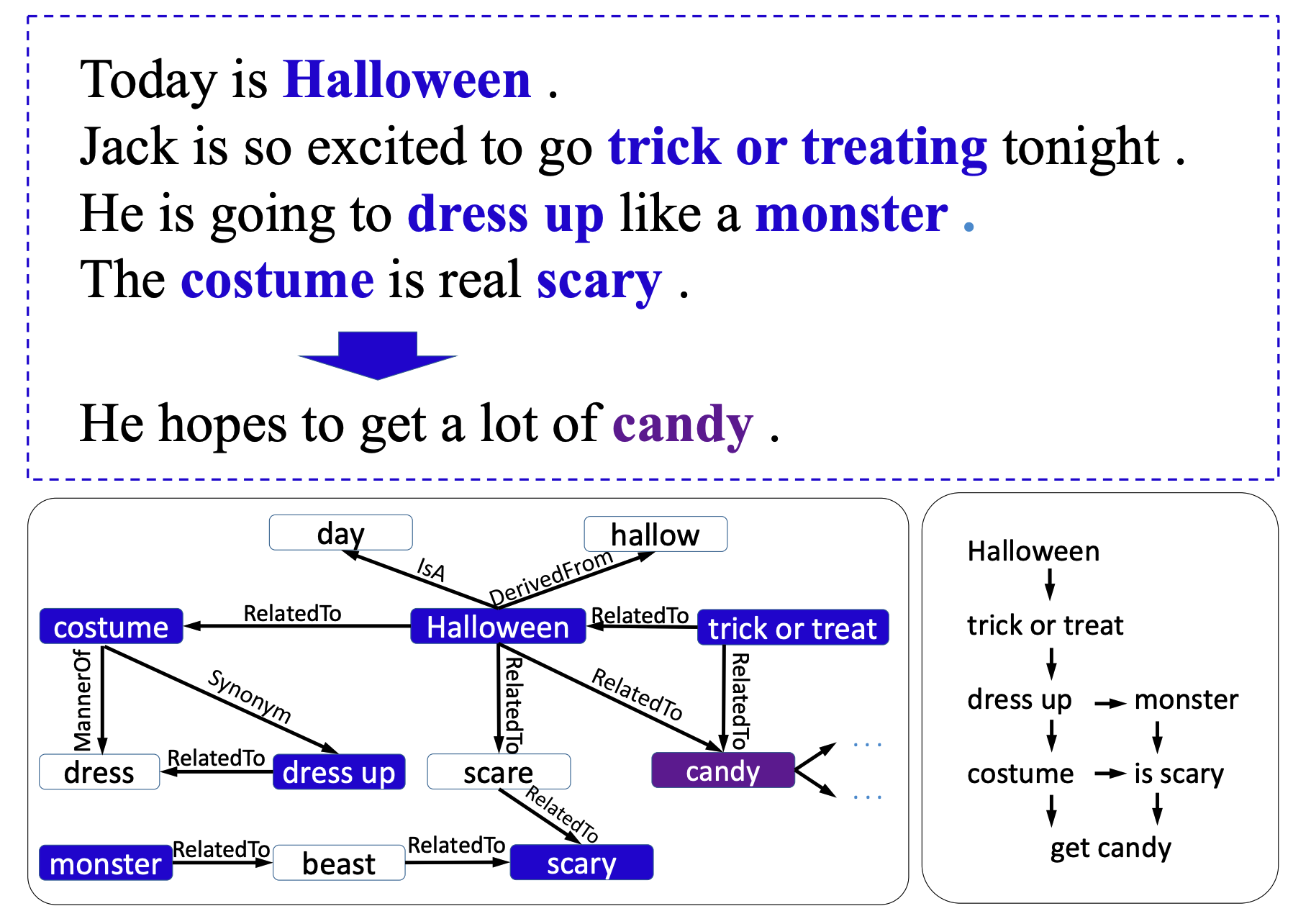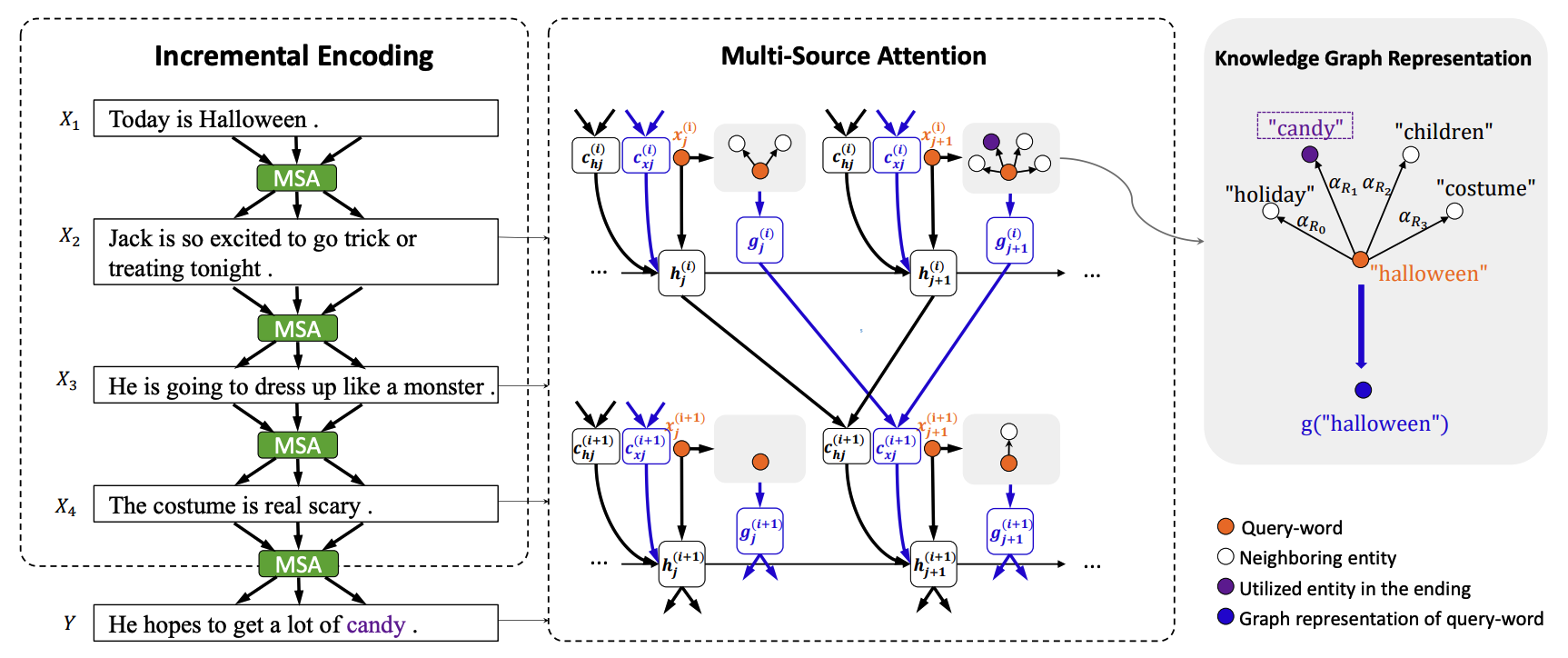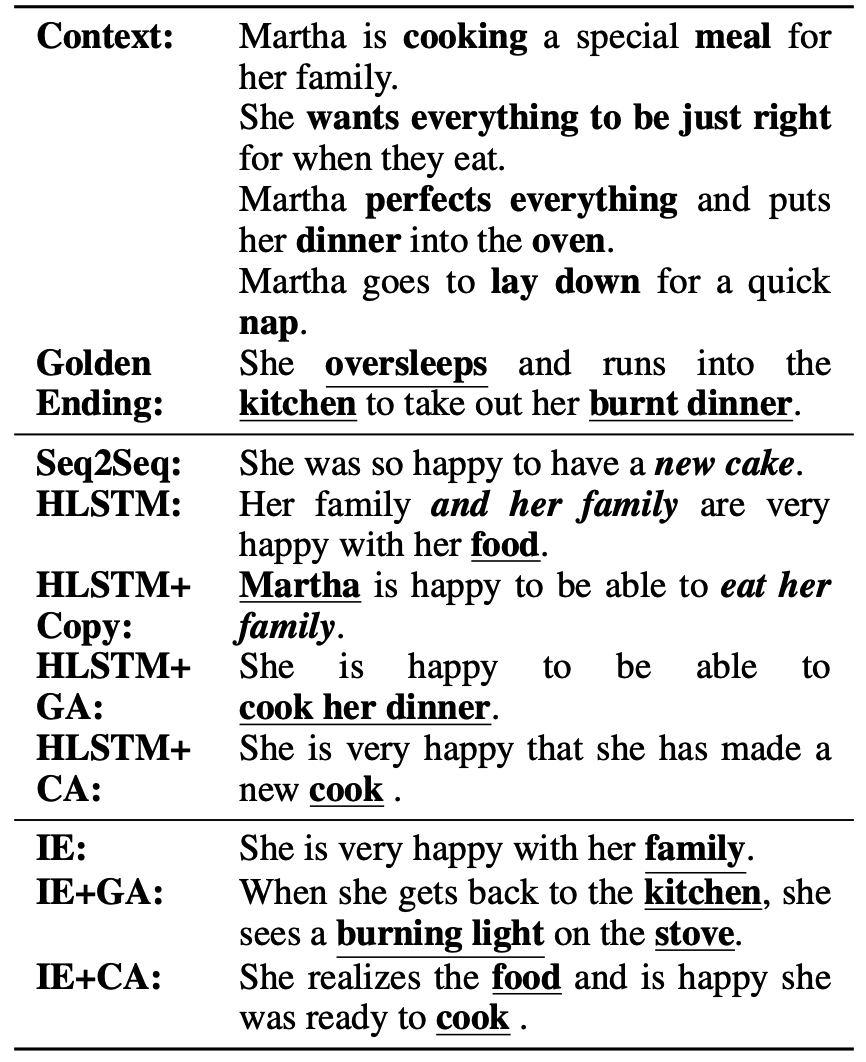# Story Ending Generation with Incremental Encoding and Commonsense Knowledge

## WHY?

Commonsense knowledge graph can be useful source of explicit knowledge for generating texts that make sense. However, it is hard to use KG since it would hold huge amount of information than needed. Retrieving graphs which is relevent for the generation is the key.

## WHAT?

The story ending generation is generating the last sentence given few lines of story.This paper bypasses the problem of graph retrieval by retrieving one-hop knowledge graph of every words in previous sentences in advance. And the model uses retrieved graphs to generate final sentences. Therefore, the task is simplified to generating plausible sentence given graphs similar to that of GraphWriter.The main two contribution of this paper is incremental encoding and multi-source attention. Incremental encoding is used to encode texts with hierarchical structure(multiple sentences).

\mathbf{h}_j^{(i)} = \mathbf{LSTM}(\mathbf{h}_{j-1}^{(i)}, \mathbf{e}(x_j^{(i)}), \mathbf{c}_{\mathbf{l}j}^{(i)}), i \geq 2

While i indexes sentence and j indexes word, \mathbf{c}_{\mathbf{l}j}^{(i)}$\mathbf{c}_{\mathbf{l}j}^{(i)}$ represent a context vector. This context vector is derived by multi-Source attention.

\mathbf{c}_{\mathbf{l}j}^{(i)} = \mathbf{W}_{\mathbf{l}}([\mathbf{c}_{\mathbf{h}j}^{(i)};\mathbf{c}_{\mathbf{x}j}^{(i)}])

\mathbf{c}_{\mathbf{h}j}^{(i)}$\mathbf{c}_{\mathbf{h}j}^{(i)}$ is derived by attending on hidden states of previous sentence, and \mathbf{c}_{\mathbf{h}j}^{(i)}$\mathbf{c}_{\mathbf{h}j}^{(i)}$ by attending on one-hop knowledge graphs of previous sentence.

This model compared two methods(graph attention and contextual attention-BiGRU) for graph representation and imposed NLL loss on generation of all sentences including previous sentences.

## So?This model generated natural sentence that makes sense using information from knowledge graph.

## Review

Retrieving all the relations related to sentences beforehand is obviously limited but effective idea. This can be good first trial to using commonsense knowledge graph for text generation.

Powered by aiden